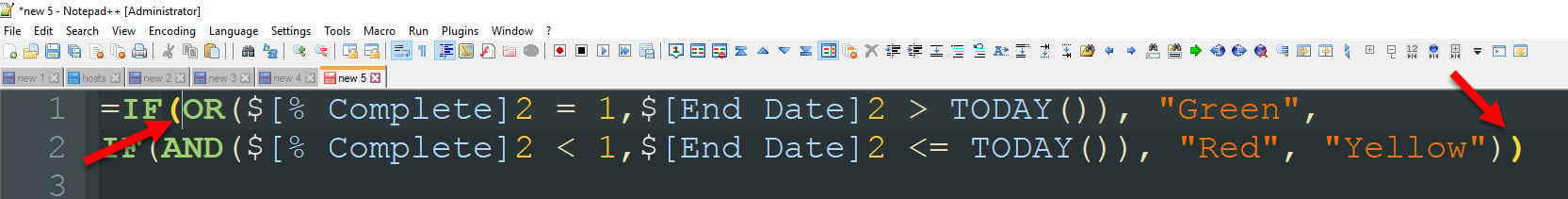or Explore Discussions

#### Welcome to the Smartsheet Forum Archives

The posts in this forum are no longer monitored for accuracy and their content may no longer be current. If there's a discussion here that interests you and you'd like to find (or create) a more current version, please Visit the Current Forums.

# How to automate RYG balls using two different column values.

11/28/17 Edited 12/09/19

I am attempting to automate the RYG balls using two different columns, the End Date and % Complete columns. The logic is as follows:

1. If the end date is greater than today, the RYG ball = Green IF([End date]2 > TODAY(), "Green"

2. If the % complete is greater than .99, the RYG ball = Green IF([% Complete]2 > .99, "Green"

3. if the % complete is empty/blank, the RYG ball = Yellow IF([% Complete]2 = "Empty", "Yellow"

4. Anything else is red

I have tried the following formulas to no avail. Any suggestions?

=IF(OR([End Date]400 > TODAY(), [% Complete]400 > 0.99, "Green")), IF([End Date]400 = Blank, "Yellow", "Red")

=IF(OR(End Date > TODAY(), [% Complete]2 = "Full"), "Green", IF(ISBLANK([End Date]2 , "Yellow", "Red"))

• Hi Jawanza,

IF(OR(\$[% Complete]2 = 1,\$[End Date]2 > TODAY()), "Green",

If your task is not done and the due date has past then Red, otherwise Yellow:

IF(AND(\$[% Complete]2 < 1,\$[End Date]2 <= TODAY()), "Red", "Yellow"))

All together:

=IF(OR(\$[% Complete]2 = 1,\$[End Date]2 > TODAY()), "Green",

IF(AND(\$[% Complete]2 < 1,\$[End Date]2 <= TODAY()), "Red", "Yellow"))

I strongly suggest a text editor like Notepad++. Use Visual Basic (VBA) as the language.

Good Luck!  (:This discussion has been closed.